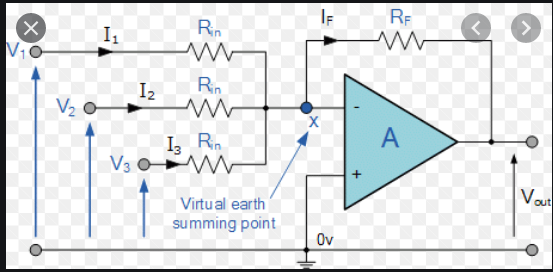This thread has been locked.

If you have a related question, please click the "Ask a related question" button in the top right corner. The newly created question will be automatically linked to this question.

# Analog Engineers Calculator

Hello ALL,

I am looking for a voltage level shifter which shifts (+1.5V to -1.5V AC signal) to (0 to +3V AC signal) for giving it to a micro-controller analog pins. I selected a simple Inverting voltage summer as shown below which serves the purpose.But, the TI's Analog Engineers calculator recommends another circuit where it asks the reference voltage to be inserted which is a non-standard value to be given at +ve pin of opamp.I would like to know whether I can go with the circuit I selected or Is there any strong rationale to use the TI recommended circuit.

• Hi Rajesh,

The Vref voltage can be created using a voltage divider and the supply voltage of the op amp. For example, if you have a 5V supply for the op amp, divide down the 5V to give you 0.65V.

Thank you,

Tim Claycomb

• Hi Tim,

Thank you for your reply.

I'd like to know the advantage of the TI Analog Engineer's Calculator recommended circuit to the classical circuit I mentioned in the original question.

• Hi Rajesh,

The main difference is that there is a reference voltage to bias up the output voltage in the TI Analog Engineer's Calculator. Without a bias voltage the output will not be able to swing from 0V to 3V.

It also appears that the classical circuit is more of a summing amplifier with V1, V2, and V3 as input voltages whereas the TI circuit is a level shifting circuit.

Thank you,

Tim Claycomb